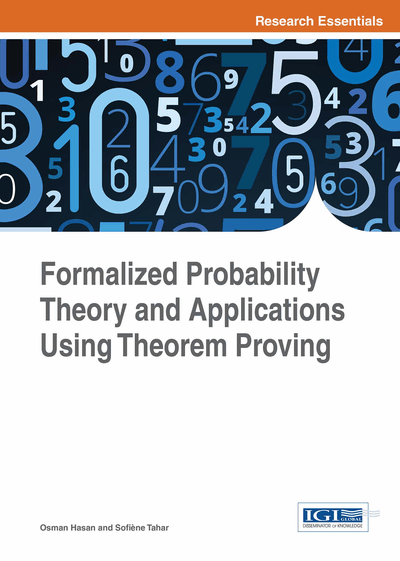# Probability Theory

DOI: 10.4018/978-1-4666-8315-0.ch005
Available
\$37.50
No Current Special Offers

## Abstract

In this chapter, the authors make use of the formalizations of measure theory and Lebesgue integration in HOL4 to provide a higher-order-logic formalization of probability theory (Mhamdi, 2013). For illustration purposes, they also present the probabilistic analysis of the Heavy Hitter problem using HOL.
Chapter Preview
Top

## 5.1 Formalization Of Probability Theory

The classical approach to define the probability of an event A is p (A) = NA / N, where NA is the number of outcomes favorable to the event A and N is the number of all possible outcomes of the experiment. However, this approach is based on the assumptions that all outcomes are equally likely (equiprobable) and that the number of possible outcomes is finite. These assumptions are not always true and thus the above-mentioned classical definition has a limited scope.

Kolmogorov introduced the axiomatic definition of probability, which overcomes the above-mentioned problem and provides a mathematically consistent way for assigning and deducing probabilities of events. This approach consists in defining a set of all possible outcomes, , called the sample space, a set F of events which are subsets of and a probability measure p such that (Ω, F, p) is a measure space with p (Ω) = 1. Now, (Ω, F, p) is a probability space if it is a measure space and p (Ω) = 1.

• Definition 5.1

`Ⱶ∀p. prob_space pmeasure_space p Ʌ (measure p (p_space p) = 1)`

A probability measure is a measure function and an event is a measurable set.

```Ⱶprob = measure
Ⱶevents = measurable_sets
Ⱶp_space = m_space```

Two events A and B are independent iff p (A ∩ B) = p (A) p (B).

• Definition 5.2

```Ⱶ ∀p a b. indep p a baevents p Ʌbevents p Ʌprob p (ab) = prob p a * prob p b
X:Ω → R is a random variable iff X is (F, B (R)) measurable```
• Definition 5.3

`Ⱶ∀ X p s. random_variable X p sprob_space p ɅXmeasurable (p_space p,events p) s`

In this book, we focus on real-valued random variables but the definition can be adapted for random variables having values on any topological space thanks to the general definition of the Borel sigma algebra.

All the properties, presented in the previous chapter, for measurable functions are obviously valid for random variables.

• Theorem 5.1

```If X and Y are random variables and c ϵ R then the following functions are also random variables: cX, | X |, Xn, X + Y, XY and max (X, Y).
Two random variables X and Y are independent iff ∀A, B ϵ B (R), the events { X ϵ A } and { Y ϵ B } are independent. The set { X ϵ A } denotes the set of outcomes ω for which X(ω) ϵ A. In other words{ X ϵ A } = X-1(A).```

## Complete Chapter List

Search this Book:
Reset